# Google Code Jam Archive — Round 2 2022 problems

## Overview

Code Jam's 2022 Round 2 was a different kind of challenge, with no easy points on the table. The first problem, Spiraling Into Control had competitors taking shortcuts through a strange house with plenty of rooms. Some clever observations could be made that help solve this problem. Next was Pixelated Circle which required some math and proofs to quickly identify missing pixels in an incorrect implementation of a 2D circle-drawing program. Saving the Jelly followed and required some matching and graph algorithms to help save Mr. Jolly's favorite candy. Finally, I, O Bot rounded out the set with contestants helping a robot cleanup giant beachballs after a giant moon party.

Um_nik was the fastest to a perfect score at 1 hour and 46 minutes, and with no penalty attempts, which assured them the top position. Benq followed just a few minutes behind, also solving all four problems on their first try. djq_cpp rounded out the top 3 by solving all four problems with few enough penalty attempts to secure their 3rd place position. In the end, over 3000 contestants got a positive number of points. The preliminary cutoff to advance to Round 3 is 25 points and a low enough penalty.

The results will be reviewed in the coming days. Those who are in the top 1000 after the round is finalized will advance to the last elimination round of the year! There are 3 weeks to get ready for Round 3 (see the schedule for details). You can practice with any of our past problems from the archive.

For those of you for whom this round is the end of the season, we hope you are very proud of making it this far. We are thrilled to see the problem solving skills of this community improve year after year. You can see a certificate highlighting your accomplishment in your profile page.

Cast

Spiraling Into Control: Written and prepared by Ian Tullis.

Pixelated Circle: Written by Archie Pusaka. Prepared by Chun-nien Chan.

Saving the Jelly: Written by Onufry Wojtaszczyk. Prepared by Nour Yosri and Yossi Matsumoto.

I, O Bot: Written by Anurag Singh. Prepared by Rahul Goswami.

Solutions and other problem preparation and review by Akul Siddalingaswamy, Alan Lou, Alex Szeto, Anson Ho, Anurag Singh, Archie Pusaka, Arjun Sanjeev, Bartosz Kostka, Bohdan Pryshchenko, Chu-ling Ko, Chun-nien Chan, Darcy Best, Devansh Gautam, Gil Vegliach, Ian Tullis, Ikumi Hide, Kevin Tran, Krists Boitmanis, Md Mahbubul Hasan, Mohamed Yosri Ahmed, Nafis Sadique, Nour Yosri, Onufry Wojtaszczyk, Pablo Heiber, Rahul Goswami, Ruoyu Zhang, S Jayasurya, and Timothy Buzzelli.

Analysis authors:

• Spiraling Into Control: Ian Tullis.
• Pixelated Circle: Chun-nien Chan.
• Saving the Jelly: Kevin Tran.
• I, O Bot: Krists Boitmanis.

## A. Spiraling Into Control

### Problem

As punishment for being naughty, Dante has been trapped in a strange house with many rooms. The house is an $$\mathbf{N} \times \mathbf{N}$$$grid of rooms, with $$\mathbf{N}$$$ odd and greater than $$1$$$. The upper left room is numbered $$1$$$, and then the other rooms are numbered $$2$$$, $$3$$$, ..., $$\mathbf{N}^2$$$, in a clockwise spiral pattern. That is, the numbering proceeds along the top row of the grid and then makes a 90 degree turn to the right whenever a grid boundary or an already numbered room is encountered, and finishes in the central room of the grid. Because $$\mathbf{N}$$$ is odd, there is always a room in the exact center of the house, and it is always numbered $$\mathbf{N}^2$$$. For example, here are the room numberings for houses with $$\mathbf{N} = 3$$$ and $$\mathbf{N} = 5$$$: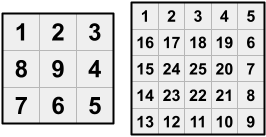Dante starts off in room $$1$$$ and is trying to reach the central room (room $$\mathbf{N}^2$$$). Throughout his journey, he can only make moves from his current room to higher-numbered, adjacent rooms. (Two rooms must share an edge — not just a corner — to be adjacent.) Dante knows that he could walk from room to room in consecutive numerical order — i.e., if he is currently in room $$x$$$, he would move to room $$x+1$$$, and so on. This would take him exactly $$\mathbf{N}^2 - 1$$$ moves. But Dante wants to do things his way! Specifically, he wants to reach the central room in exactly $$\mathbf{K}$$$moves, for some $$\mathbf{K}$$$ strictly less than $$\mathbf{N}^2 - 1$$$. Dante can accomplish this by taking one or more shortcuts. A shortcut is a move between rooms that are not consecutively numbered. For example, in the $$5 \times 5$$$ house above,

• If Dante is at $$1$$$, he cannot move to $$17$$$, but he can move to $$2$$$or to $$16$$$. The move to $$2$$$is not a shortcut, since $$1 + 1 = 2$$$. The move to $$16$$$is a shortcut, since $$1 + 1 \neq 16$$$.
• From $$2$$$, it is possible to move to $$3$$$ (not a shortcut) or to $$17$$$(a shortcut), but not to $$1$$$, $$16$$$, or $$18$$$.
• From $$24$$$, Dante can only move to $$25$$$ (not a shortcut).
• It is not possible to move out of room $$25$$$. As a specific example using the $$5 \times 5$$$ house above, suppose that $$\mathbf{K}$$$= $$4$$$. One option is for Dante to move from $$1$$$to $$2$$$, then move from $$2$$$to $$17$$$ (which is a shortcut), then move from $$17$$$to $$18$$$, then move from $$18$$$to $$25$$$ (which is another shortcut). This is illustrated below (the red arrows represent shortcuts):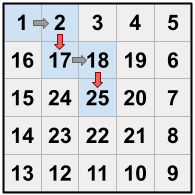Can you help Dante find a sequence of exactly $$\mathbf{K}$$$moves that gets him to the central room, or tell him that it is impossible? ### Input The first line of the input gives the number of test cases, $$\mathbf{T}$$$. $$\mathbf{T}$$$test cases follow. Each test case consists of one line with two integers $$\mathbf{N}$$$ and $$\mathbf{K}$$$, where $$\mathbf{N}$$$ is the dimension of the house (i.e. the number of rows of rooms, which is the same as the number of columns of rooms), and $$\mathbf{K}$$$is the exact number of moves that Dante wants to make while traveling from room $$1$$$ to room $$\mathbf{N}^2$$$. ### Output For each test case, output one line containing Case #$$x$$$: $$y$$$, where $$x$$$ is the test case number (starting from 1).

If no valid sequence of exactly $$\mathbf{K}$$$moves will get Dante to the central room, $$y$$$ must be IMPOSSIBLE.

Otherwise, $$y$$$must be an integer: the number of times that Dante takes a shortcut, as described above. (Notice that because Dante wants to finish in strictly less than $$\mathbf{N}^2 - 1$$$ moves, he must always use at least one shortcut.) Then, output $$y$$$more lines of two integers each. The $$i$$$-th of these lines represents the $$i$$$-th time in Dante's journey that he takes a shortcut, i.e., he moves from some room $$a_i$$$ to another room $$b_i$$$such that $$a_i + 1 \lt b_i$$$.

Notice that because these lines follow the order of the journey, $$a_i \lt a_{i+1}$$$for all $$1 \le i \lt y$$$.

### Limits

Memory limit: 1 GB.
$$1 \le \mathbf{T} \le 100$$$. $$1 \le \mathbf{K} \lt \mathbf{N}^2 - 1$$$.
$$\mathbf{N} \mod 2 \equiv 1$$$. ($$\mathbf{N}$$$ is odd.)

#### Test Set 1 (Visible Verdict)

Time limit: 5 seconds.

#### Test Set 3 (Hidden Verdict)

Time limit: 20 seconds.
$$3 \le \mathbf{N} \le 9999$$$. ### Sample Sample Input 4 5 4 5 3 5 12 3 1  Sample Output Case #1: 2 2 17 18 25 Case #2: IMPOSSIBLE Case #3: 2 11 22 22 25 Case #4: IMPOSSIBLE  Sample Case #1 is described in the problem statement. Dante's route is $$1 \to 2 \to 17 \to 18 \to 25$$$. Because $$1 \to 2$$$and $$17 \to 18$$$ are moves between consecutively numbered rooms, they are not included in the output. Only the shortcuts ($$2 \to 17$$$and $$18 \to 25$$$) are included.

In Sample Case #2, there is no solution. (Recall that there is no way for Dante to move diagonally.)

In Sample Case #3, observe that $$22$$$appears both as the end of one shortcut and the start of the next. It would not be valid to include the line 11 22 25 in the output; each line must represent a single shortcut.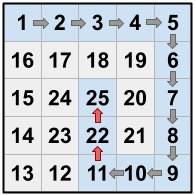There is another solution that uses only one shortcut: Dante can move from $$1 \to 2 \to 3 \to 4 \to 5 \to 6$$$, then move from $$6 \to 19$$$(a shortcut), then move from $$19 \to 20 \to 21 \to 22 \to 23 \to 24 \to 25$$$. This is also valid; there is no requirement to minimize (or maximize) the number of shortcuts taken.

In Sample Case #4, Dante cannot get to the central room ($$9$$$, in this case) in just one move. ## B. Pixelated Circle ### Problem Typical computer images are matrices of pixels, with each pixel being a small square of a specific color. Drawing lines that are not perfectly parallel to the axes of the pixel matrix results in imperfections. Drawing circles is an extreme example where those imperfections arise. Suppose we have a picture consisting of $$2\mathbf{R}+1$$$ by $$2\mathbf{R}+1$$$pixels, and we number the rows and columns of pixels between $$-\mathbf{R}$$$ and $$\mathbf{R}$$$, such that the center pixel is at row $$0$$$ and column $$0$$$. Initially, all pixels are white. Then, a circle of radius $$\mathbf{R}$$$ and centered in the picture can be drawn in black by the following pseudocode, where set_pixel_to_black(x, y) makes the pixel at row $$x$$$and column $$y$$$ be colored black.

draw_circle_perimeter(R):
for x between -R and R, inclusive {
y = round(sqrt(R * R - x * x))   # round to nearest integer, breaking ties towards zero
set_pixel_to_black(x, y)
set_pixel_to_black(x, -y)
set_pixel_to_black(y, x)
set_pixel_to_black(-y, x)
}


Notice that some pixels may be set to black more than once by the code, but the operation is idempotent (that is, calling set_pixel_to_black on a pixel that is already black changes nothing).

The following is pseudocode for a function to draw a filled circle (starting from an all-white picture).

draw_circle_filled(R):
for x between -R and R, inclusive {
for y between -R and R, inclusive {
if round(sqrt(x * x + y * y)) <= R:
set_pixel_to_black(x, y)
}
}


And finally, the following is pseudocode to incorrectly draw a filled circle:

draw_circle_filled_wrong(R):
for r between 0 and R, inclusive {
draw_circle_perimeter(r)
}


### Output

For each test case, output one line containing Case #$$x$$$: $$y$$$, where $$x$$$is the test case number (starting from 1) and $$y$$$ is the number of pixels that would have different colors between a picture in which draw_circle_filled($$\mathbf{R}$$$) is called and another one in which draw_circle_filled_wrong($$\mathbf{R}$$$) is called.

### Limits

Memory limit: 1 GB.

#### Test Set 2 (Hidden Verdict)

Time limit: 15 seconds.
$$1 \le \mathbf{R} \le 10^5$$$. ### Sample Sample Input 3 2 8 50  Sample Output Case #1: 4 Case #2: 24 Case #3: 812  In Sample Case #1, 21 pixels are drawn in black by calling draw_circle_filled(2) (shown in the left picture). 17 pixels are drawn in black by calling draw_circle_filled_wrong(2) (shown in the right picture). Four pixels would have different colors between the two pictures: $$(-1, -1)$$$, $$(-1, 1)$$$, $$(1, -1)$$$, and $$(1, 1)$$$, where $$(x, y)$$$ represents the pixel at row $$x$$$and column $$y$$$, with the rows and columns numbered as described in the statement.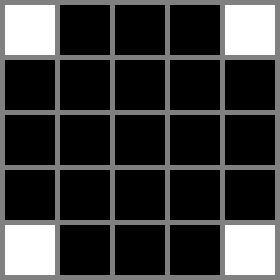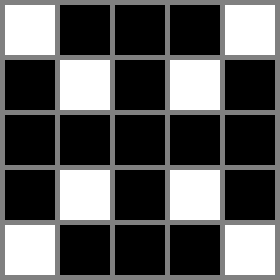In Sample Case #2, the following pictures are the images generated by calling draw_circle_filled(8) (left) and draw_circle_filled_wrong(8) (right).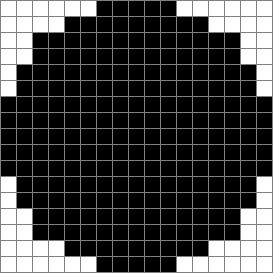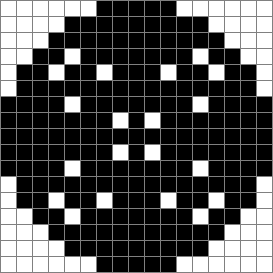## C. Saving the Jelly

### Problem

Mr. Jolly teaches football (or soccer, for US speakers) to $$\mathbf{N}$$$children numbered from $$1$$$ to $$\mathbf{N}$$$. He has taken to leaving sweets on the field where the games take place, one for each child. After the game is finished, each child can grab and eat one sweet as their reward. The children are tired after games, so each child wants to grab the sweet closest to them (using Euclidean distance). This could lead to fights — if the same sweet is closest to two or more children. To avoid that, after the game all the children stop where they are, and Mr. Jolly calls out their names, one by one. When a child's name is called, they grab the closest sweet to them (out of the ones that weren't already grabbed, of course). In the case where two or more sweets are tied for the smallest distance, Mr. Jolly can decide which one the child grabs.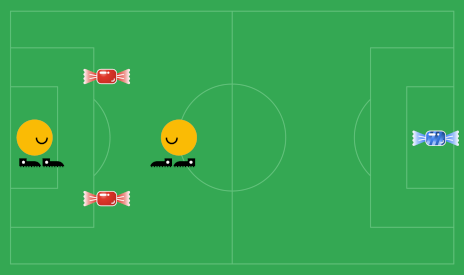This has worked very well for Mr. Jolly for a while now, but today disaster struck! While laying out the sweets, Mr. Jolly accidentally dropped his blueberry jelly that he planned to eat after all the children go home. So now there are $$\mathbf{N}$$$ children on the field, and $$\mathbf{N}+1$$$sweets. The sweets are numbered from $$1$$$ to $$\mathbf{N} + 1$$$, with sweet $$1$$$ being Mr. Jolly's blueberry jelly. Is there a way for Mr. Jolly to save his blueberry jelly by calling the children's names in such an order that the blueberry jelly is the one sweet left over?

### Limits

Memory limit: 1 GB.

#### Test Set 2 (Hidden Verdict)

Time limit: 45 seconds.
$$1 \le \mathbf{N} \le 1000$$$. ### Sample Sample Input 4 2 -3 0 -1 0 3 0 -2 -1 -2 1 1 0 0 1 1 2 2 3 10 0 -10 0 0 0 0 5 -1 0 5 0 0 -5 2 3 4 3 4 5 7 3 4 5 7  Sample Output Case #1: POSSIBLE 2 2 1 3 Case #2: IMPOSSIBLE Case #3: POSSIBLE 3 2 2 4 1 3 Case #4: POSSIBLE 1 2 2 3  Sample Case #1 is illustrated in the image above. Notice that each child is equally close to each of the two non-blueberry-jelly sweets. In our solution, Mr. Jolly assigns the second sweet to the second child and the third sweet to the first child, successfully leaving the first sweet (the blueberry jelly) for himself. In Sample Case #2, the sole child is closer to the blueberry jelly than to the other sweet, so Mr. Jolly cannot prevent his precious blueberry jelly from being eaten. In Sample Case #3, we present one of many solutions; it is actually possible to call the children in any order. In Sample Case #4, note that children might share the same position, sweets might share the same position, and children and sweets might share the same position. ## D. I, O Bot ### Problem To welcome attendees to a developers' conference on Jupiter's moon of Io, the organizers inflated many giant beach balls. Each ball is in roughly the shape of either a $$1$$$ or a $$0$$$, since those look sort of like the letters I and O. The conference just ended, and so now the beach balls need to be cleaned up. Luckily, the beach ball cleanup robot, BALL-E, is on the job! The conference was held on an infinite horizontal line, with station $$0$$$ in the middle, stations $$1, 2, \dots$$$to the right, and stations $$-1, -2, \dots$$$ to the left. Station 0 contains the conference's only beach ball storage warehouse. Each other station contains at most one beach ball.BALL-E has two storage compartments, each of which can hold a single beach ball. One compartment can only hold $$1$$$⁠-shaped balls and the other can only hold $$0$$$⁠-shaped balls. (The $$1$$$⁠-shaped balls are more oblong than the $$0$$$⁠-shaped balls, so neither shape of ball will fit in the other shape's compartment.)

BALL-E initially has both the $$0$$$and $$1$$$ compartments empty, and it starts off at station $$0$$$. The robot can do the following things: • Move left one station or right one station. This costs 1 unit of power. • If there is a ball at the current station, and BALL-E is not already storing a ball of that shape, it can put the ball in the appropriate compartment. This takes 0 units of power. • If there is a ball at the current station, BALL-E can compress it so that its shape becomes the other shape. That is, a $$1$$$⁠-shaped ball becomes a $$0$$$⁠-shaped ball, or vice versa. It takes $$\mathbf{C}$$$ units of power to do this. Note that BALL-E may not change the shape of a ball that it has already put into one of its compartments.
• If BALL-E is at station 0 and is storing at least one ball, it can deposit all balls from its compartment(s) into the beach ball storage warehouse. This takes 0 units of power and leaves both compartments empty.

Notice that if BALL-E moves to a station and there is a ball there, BALL-E is not required to pick it up immediately, even if the robot has an open compartment for it. Also, if BALL-E moves to the station with the warehouse, it is not required to deposit any balls it has.

Find the minimum number of units of power needed for BALL-E to transfer all of the balls to the warehouse, using only the moves described above.

### Input

The first line of the input gives the number of test cases, $$\mathbf{T}$$$. $$\mathbf{T}$$$ test cases follow.
The first line of each test case contains two integers, $$\mathbf{N}$$$and $$\mathbf{C}$$$: the number of balls and the amount of power units needed to change the shape of a ball, respectively.
The next $$\mathbf{N}$$$lines describe the positions (i.e., station numbers) and the shapes of the balls. The $$i$$$-th line contains two integers, $$\mathbf{X_i}$$$and $$\mathbf{S_i}$$$: the position and the shape of the $$i$$$-th ball, respectively. ### Output For each test case, output one line containing Case #$$x$$$: $$y$$$, where $$x$$$ is the test case number (starting from 1) and $$y$$$is the minimum number of units of power needed to transfer all of the balls to the warehouse, as described above. ### Limits Time limit: 40 seconds. Memory limit: 1 GB. $$1 \le \mathbf{T} \le 100$$$.
$$0 \le \mathbf{S_i} \le 1$$$, for all $$i$$$.
$$-10^9 \le \mathbf{X_i} \le 10^9$$$, for all $$i$$$.
$$0 \le \mathbf{C} \le 10^9$$$. $$\mathbf{X_i} ≠ 0$$$, for all $$i$$$. All $$\mathbf{X_i}$$$ are distinct.

#### Test Set 1 (Visible Verdict)

For at most 15 cases:
$$1 \le \mathbf{N} \le 5000$$$. For the remaining cases: $$1 \le \mathbf{N} \le 100$$$.

#### Test Set 2 (Hidden Verdict)

For at most 15 cases:
$$1 \le \mathbf{N} \le 10^5$$$. For the remaining cases: $$1 \le \mathbf{N} \le 5000$$$.

### Sample

Sample Input
4
5 0
3 0
6 0
8 0
10 1
15 1
5 10
3 0
6 0
8 0
10 1
15 1
5 1
3 0
6 0
8 0
10 1
15 1
2 0
1000000000 0
-1000000000 1

Sample Output
Case #1: 52
Case #2: 56
Case #3: 54
Case #4: 4000000000


In Sample Case #1 (illustrated in the statement), there are $$\mathbf{N} = 5$$$balls and $$\mathbf{C} = 0$$$. One optimal strategy is to make three round trips from (and back to) the warehouse:

• First round trip: Move to station $$3$$$, pick up the $$0$$$ ball there and store it in the $$0$$$compartment, move back to station $$0$$$, and deposit the ball in the warehouse. This takes $$6$$$units of power. • Second round trip: Move to station $$8$$$, pick up the $$0$$$ball there, and store it in the $$0$$$ compartment. Move to station $$6$$$, change the $$0$$$ ball there into a $$1$$$ball, and pick it up and store it in the $$1$$$ compartment. Move to station $$0$$$and deposit both balls in the warehouse. This takes $$16$$$ units of power. (Recall that in this case, it takes $$0$$$units of power to change a ball's shape.) • Third round trip: Move to station $$10$$$. Change the $$1$$$ball there into a $$0$$$ ball, and pick it up and store it in the $$0$$$compartment. Move to station $$15$$$. Pick up the $$1$$$ball there and store it in the $$1$$$ compartment. Move to station $$0$$$and deposit both balls in the warehouse. This takes $$30$$$ units of power.

The total number of units of power needed to collect all the balls is $$52$$$. Sample Case #2 is like Sample Case #1, but now with $$\mathbf{C} = 10$$$. Now BALL-E has to use at least $$56$$$units of power: • First round trip: Get the ball from station $$3$$$. This takes $$6$$$units of power. • Second round trip: Get the differently-shaped balls from stations $$6$$$ and $$10$$$. (These are a $$0$$$ and a $$1$$$, respectively, so there is no need to change the shape of either of them.) This takes $$20$$$ units of power.
• Third round trip: Get the differently-shaped balls from stations $$8$$$and $$15$$$. This takes $$30$$$units of power. Sample Case #3 is also like Sample Case #1, but now with $$\mathbf{C} = 1$$$. Here, BALL-E needs at least $$54$$$units of power: • First round trip: Get the ball from station $$3$$$. This takes $$6$$$units of power. • Second round trip: Get the ball from station $$8$$$. When passing through station $$6$$$on the way back, change the shape of the ball there and get it. This takes $$17$$$ units of power.
• Third round trip: Do the same with the balls at stations $$15$$$and $$10$$$. This takes $$31$$$units of power. In Sample Case #4, one optimal strategy is for BALL-E to move to station $$-1000000000$$$, get the $$1$$$ball there, move to station $$1000000000$$$, get the $$0$$$ball there, and then return to station $$0$$$ to deposit both of them.

## Analysis — A. Spiraling Into Control

### Test Sets 1 and 2

You may or may not have run across the old chestnut of a technical interview question that asks you to number all of the cells of a grid in a spiral pattern. In this problem, doing that is potentially helpful for the first two test sets. However, the last test set can be (and must be) solved without explicitly creating and numbering a spiral. (For a case with $$\mathbf{N} = 9999$$$, we would need to store and number almost $$10^8$$$ cells!) We'll worry about that later in the analysis.

To make a numbered spiral, we can create a grid and loop through it, starting from the upper left cell, and making a 90 degree turn to the right each time we encounter a grid boundary or an already-numbered cell. One clean way to do this is to use an array of "directions": $$((0, 1), (1, 0), (0, -1), (-1, 0))$$$, where the starting direction $$(0, 1)$$$ means stay in the same row and move one column right, and so on, with the other three referring to moves downward, leftward, and upward, respectively, in the grid. When our current direction is $$(\Delta r, \Delta c)$$$and we are at cell $$(r, c)$$$ in the grid, we check cell $$(r + \Delta r, c + \Delta c)$$$to see if it is outside the grid or has already been numbered. If so, we choose the next direction in the direction vector, looping back to the start if we go off the end, and then calculate $$(r + \Delta r, c + \Delta c)$$$ using the new $$(\Delta r, \Delta c)$$$. Then, whether or not we changed direction, we label the current cell, increment our label counter, and proceed to the cell $$(r + \Delta r, c + \Delta c)$$$. We stop when we label the $$\mathbf{N}^2$$$-th cell. For Test Set 1, we can exhaustively enumerate all legal paths, using our spiral numbering to determine which moves are allowed. We can keep track of the shortcuts taken in each possible path, and see whether any path finishes in exactly $$\mathbf{K}$$$ moves. Since there is a shortcut to take (or not take) in almost every cell, this solution is exponential.

For Test Set 2, we can refine this idea to avoid explicitly considering all possible paths. For each cell in the grid, we create an array that can hold up to one path for every possible number of moves "so far". Then we proceed along the spiral in consecutive numerical order. For every cell we visit, for every path in that cell's array, we try to extend it into all legal neighboring cells.

For example, in a $$5 \times 5$$$grid, we start at cell 1 with only a way to get there in 0 moves. We tell each of the legal neighboring cells (2 and 16) that we have a way to get there in 1 move, starting from cell 1. Then, in cell 2, we tell each of cells 3 and 17 that we have a way to get there in 2 moves, with the starting prefix $$1, 2$$$, and so on. The key difference from the Test Set 1 solution is that when a cell is offered a path and it already has a path with exactly that number of moves, it does not store the new path. This cuts down on the proliferation of possible paths.

And so we have a dynamic programming / memoization solution. Since there is at most one shortcut to take in each cell, we do constant work per cell; since we are populating a table that is $$\mathbf{N}^2 \times \mathbf{N}$$$, this solution takes $$O(\mathbf{N}^3)$$$ time.

### Test Set 3

To be able to handle grids with $$\mathbf{N}$$$up to 9999, we need to do three things efficiently: • Given a value of $$\mathbf{K}$$$, determine whether a path with exactly $$\mathbf{K}$$$moves exists. • Find such a path. • Given the coordinates of a grid cell, find its number in the spiral. We can begin by observing that the following values of $$\mathbf{K}$$$ are IMPOSSIBLE:

1. $$\mathbf{K} \lt \mathbf{N}-1$$$. In this case, even if we move as directly as possible toward the center (taking only shortcuts), there are not enough moves to get there. 2. Odd $$\mathbf{K}$$$. We can see this via a "checkerboard argument". Imagine that the grid has a checkerboard pattern, with the upper left cell being black. Then the diagonal running from the upper left cell to the lower right cell is entirely black, and so the central cell is black. Now, each move is in an orthogonal (i.e., "compass") direction, so each move either takes us from a black cell to a red cell or vice versa. Therefore, to end on the central cell, which is black, we must make an even number of moves.

As it turns out, these are the only impossible cases! To see why, it helps to think of the spiral as consisting of concentric square rings, with "ring" 0 being just the central cell, ring 1 being the eight cells around that, ring 2 being the sixteen cells around ring 1, and so on. For example, the rings in the $$\mathbf{N} = 7$$$grid look like this:  3333333 3222223 3211123 3210123 3211123 3222223 3333333  In ring 1, there are three possible shortcuts: we can move into ring 0 from the top, right, or bottom, and this will save us 6, 4, or 2 moves, respectively, compared to just walking through all of ring 1 (and into ring 0) without taking a shortcut. But we can only take one of these shortcuts. In ring 2 (and beyond), for simplicity, let's only consider taking shortcuts that are in the same row or column as the central cell. There are four such shortcuts, and they will save us 14, 12, 10, or 8 moves, respectively. Notice that if we take the 8-move shortcut from ring 2, for example, we cannot use any of the shortcuts in ring 1. However, if we take the 14-move shortcut from ring 2, we will still have access to all three shortcuts in ring 1. Similarly, in ring 3, the shortcuts save us 22, 20, 18, or 16 moves, and if we take the first of these, we still have access to all four shortcuts in ring 2. We can turn these observations into a constructive solution for any even $$\mathbf{K}$$$ that has an answer:

• If we want to save between 2 and 22 moves, we take the specific shortcut with that savings.
• If we want to save between 24 and 36 moves, we can take the 22-move shortcut and then take the specific shortcut (from ring 1 or 2) that gets us the remaining savings.
• If we want to save 38, 40, or 42 moves, we can take the 22-move and 14-move shortcuts and then the 2, 4, or 6-move shortcut from ring 1.
• We already established that we cannot save 44 moves or more (since then $$\mathbf{K}$$$would be less than $$\mathbf{N}-1$$$).

Generalizing this strategy: we can find the number of moves we need to save (which is $$\mathbf{N}^2 - 1 - \mathbf{K}$$$; call it $$s$$$), and then proceed from the outermost ring to the innermost. At each ring $$r$$$, 1. If $$s$$$ is greater than or equal to the number of moves saved by the largest shortcut (i.e., $$8r - 2$$$moves), we take that shortcut, subtract its savings from $$s$$$, and proceed to the $$r-1$$$-th ring. 2. If $$s$$$ is equal to the number of moves saved by some other shortcut in ring $$r$$$(i.e., $$8r - 4$$$, $$8r - 6$$$, or $$8r - 8$$$), we take that shortcut and stop. (We must be careful not to do this when $$8r - 8 = 0$$$, since that move — from cell $$\mathbf{N}^2 - 1$$$ to cell $$\mathbf{N}^2$$$— saves us nothing and is not a shortcut! 3. Otherwise, we reject all shortcuts in ring $$r$$$, and proceed to ring $$r-1$$$. All that remains is to find the room numbers for the shortcuts that we take. Instead of trying to generate the entire spiral, we can notice that, say, the upper left corners of the rings (starting in the central cell and going outward) have values $$\mathbf{N}^2, \mathbf{N}^2 - 8, \mathbf{N}^2 - 8 - 16, \mathbf{N}^2 - 8 - 16 - 24,$$$ etc. We can even turn this into a formula: the upper left cell of ring $$r$$$has number $$\mathbf{N}^2 - 8 \sum_{i=0}^r i = \mathbf{N}^2 - 4(r)(r+1)$$$.

Once we have the number of the upper left cell of a ring, it is not too hard to find the values of the cells we are using for shortcuts in that ring (i.e., the ones in the central row or column). If the upper left cell's number is $$x$$$, the shortcuts have numbers $$x+r, x+3r, x+5r, x+7r$$$.

Because there are $$\frac{\mathbf{N}+1}{2}$$$rings in total, and we do $$O(1)$$$ work in each of them, this solution is $$O(\mathbf{N})$$$and easily passes Test Set 3. There are values of $$\mathbf{K}$$$ that require a shortcut to be used in every ring (except the central cell), so we can't do better than this in general.

### It could have been worse...

We thought about presenting this problem in a different way, without mentioning spirals: starting from the upper left cell of an $$\mathbf{N} \times \mathbf{N}$$$grid, give a path that reaches the central cell in exactly $$\mathbf{K}$$$ moves, or say it is impossible. One solution is to come up with the spiral path and strategy from this problem! But there were many other time-wasting roads to go down there, and the problem was already challenging for the first problem of a Round 2.

Test Data
info We recommend that you practice debugging solutions without looking at the test data.

## Analysis — B. Pixelated Circle

### Test Set 2

The key observation for optimizing the solution is that for any $$\mathbf{R}$$$, every pixel colored by draw_circle_filled_wrong($$\mathbf{R}$$$) is also colored by draw_circle_filled($$\mathbf{R}$$$), that is $$C_w \subseteq C$$$. Therefore, we can simplify the size of symmetric difference to:

$$\begin{equation}\begin{split} |C \Delta C_w| &= |(C \setminus C_w) \cup(C_w \setminus C) | \\ &= |(C \setminus C_w) \cup \emptyset | \\ &= |C| - |C\cap C_w| \\ &= |C| - |C_w| \\ \end{split}\end{equation}$$$which means we can count the number of pixels colored by draw_circle_filled($$\mathbf{R}$$$) and draw_circle_filled_wrong($$\mathbf{R}$$$) separately, and the answer would be the difference between these two numbers. The proof of this observation is given at the end of this analysis. #### Count pixels colored by draw_circle_filled($$\mathbf{R}$$$)

To get the number of pixels colored by draw_circle_filled($$\mathbf{R}$$$), we need to iterate through all possible values of $$x$$$ and find $$y_{min}$$$and $$y_{max}$$$ for each $$x$$$which satisfy $$\mathbf{round}(\sqrt{x^2 + y^2}) \le \mathbf{R}$$$ for all $$y_{min} \leq y \leq y_{max}$$$. A solution for them is $$y_{max} = \mathbf{floor}(\sqrt{\mathbf{R} + 0.5}^2 - x^2)$$$ and $$y_{min} = -y_{max}$$$. Therefore, we can get the number of colored pixels with a for-loop for the following equation: $$\begin{equation} |C| = \sum_{x=-\mathbf{R}}^{\mathbf{R}}{\mathbf{floor}(\sqrt{(\mathbf{R} + 0.5)^2 - x^2}) \times 2 + 1} \end{equation}$$$

Time complexity: $$O(\mathbf{R})$$$#### Count pixels colored by draw_circle_filled_wrong($$\mathbf{R}$$$)

draw_circle_filled_wrong($$\mathbf{R}$$$) is composed of draw_circle_perimeter($$r$$$) calls with $$r$$$from $$0$$$ to $$\mathbf{R}$$$. Notice that pixels colored by draw_circle_perimeter($$r_1$$$) and draw_circle_perimeter($$r_2$$$) never overlap if $$r_1 \neq r_2$$$. Based on this observation, we can count the number of pixels colored by each draw_circle_perimeter($$r$$$) separately and sum them up to get the total number of colored pixels. The proof of this observation is given at the end of this analysis. Looking into function draw_circle_perimeter($$r$$$), we can break the colored pixels into 4 quadrants and count them separately. Since the colored pattern is symmetric to both x-axis and y-axis, we just need to count the pixels in Quadrant 1 (Q1), and the total count excluding the origin pixel would be that number times 4, and plus 1 to include the origin pixel.

For $$r \ge 1$$$, the colored pixels in Q1 are symmetric to the line $$x=y$$$, and there are exactly $$x_t$$$colored pixels between y-axis and $$(x_t, y_t)$$$, the closest point to line $$x=y$$$(above or on the line, $$x_t \ge y_t$$$). Since $$x=y$$$is at $$45^{\circ}$$$ to the x-axis, the integer $$x_t$$$would be either $$\mathbf{ceil}(r/\mathbf{cos}(45^{\circ}))$$$ or $$\mathbf{floor}(r/\mathbf{cos}(45^{\circ}))$$$. We can compute the corresponding $$y_t = \mathbf{round}(\sqrt{r^2 - x_t^2})$$$ and choose the closer one above or on the line $$x=y$$$. Afterwards, the number of colored pixels in Q1 including x-axis would be $$2 \times x_t + 1$$$, and minus 1 if $$(x_t, y_t)$$$lies on the line $$x=y$$$ since it is not mirrored in this case.

Time complexity: $$O(1)$$$for counting pixels colored by draw_circle_perimeter($$r$$$), and $$O(\mathbf{R})$$$for all colored pixels. #### Proof: $$C_w \subseteq C$$$

For every positive $$\mathbf{R}$$$, $$r$$$ and $$x$$$such that $$0 \leq r \leq \mathbf{R}$$$ and $$-r \leq x \leq r$$$, we want to prove that the following inequality always satisfies: $$\begin{equation}\begin{split} & \mathbf{round}(\sqrt{x^2 + \mathbf{round}(\sqrt{r^2 - x^2})^2}) \leq r \\ \iff& \sqrt{x^2 + \mathbf{round}(\sqrt{r^2 - x^2})^2} - 0.5 \leq r \\ \iff& \sqrt{x^2 + \mathbf{round}(\sqrt{r^2 - x^2})^2} \leq r + 0.5 \\ \iff& x^2 + \mathbf{round}(\sqrt{r^2 - x^2})^2 \leq r^2 + r + 0.25 \\ \iff& x^2 + (\sqrt{r^2 - x^2} + 0.5)^2 \leq r^2 + r + 0.25 \\ \iff& r^2 + \sqrt{r^2 - x^2} + 0.25 \leq r^2 + r + 0.25 \\ \end{split}\end{equation}$$$

which always holds since $$\sqrt{r^2 - x^2} \leq \sqrt{r^2} = r$$$when $$|x| \leq r$$$ and $$r \ge 0$$$. Secondly, $$y = \mathbf{round}(\sqrt{r^2 - x^2}) \leq \mathbf{round}(\sqrt{r^2}) \leq \mathbf{R}$$$. Therefore $$-\mathbf{R} \leq y \leq \mathbf{R}$$$always holds. The proof above shows that pixels colored by draw_circle_filled_wrong($$r$$$) with $$0 \leq r \leq \mathbf{R}$$$also satisfy the coloring condition in draw_circle_filled($$\mathbf{R}$$$), which implies $$C_w \subseteq C$$$. #### Proof: draw_circle_perimeter($$r_1$$$) and draw_circle_perimeter($$r_2$$$) never overlap For the first coloring statement in draw_circle_perimeter($$r$$$), we want to prove that given a fixed $$x$$$, the inequality $$y_1 = \mathbf{round}(\sqrt{r_1^2 - x^2}) \neq y_2 = \mathbf{round}(\sqrt{r_2^2 - x^2})$$$ for any pair of integers $$r_1$$$and $$r_2$$$ such that $$r_1 \gt r_2 \geq 0$$$and $$|x| \leq r_2$$$ is always true:

$$\begin{equation}\begin{split} &|\sqrt{r_1^2 - x^2} - \sqrt{r_2^2 - x^2}| &\\ =\;& \sqrt{r_1^2 - x^2} - \sqrt{r_2^2 - x^2} &\; r_1 \gt r_2 \\ \geq\;& \sqrt{r_1^2 - x^2} - \sqrt{(r_1 - 1)^2 - x^2} &\; r_1 \texttt{ and } r_2 \texttt{ are integers} \\ \geq\;& (\sqrt{r_1^2} - \sqrt{x^2}) - (\sqrt{(r_1 - 1)^2} - \sqrt{x^2}) &\; \texttt{for any } |x| \leq r_1 - 1 \\ =\;& r_1 - x - (r_1 - 1) + x \\ =\;& 1 \end{split}\end{equation}$$$Based on the proof above, we can further get: $$\begin{equation}\begin{split} & \mathbf{round}(\sqrt{r_1^2 - x^2}) -\mathbf{round}(\sqrt{r_2^2 - x^2}) \\ \geq\;& \mathbf{round}(\sqrt{r_1^2 - x^2}) -\mathbf{round}(\sqrt{(r_1 - 1)^2 - x^2})\\ \geq\;& \mathbf{round}(\sqrt{r_1^2 - x^2}) - \mathbf{round}(\sqrt{r_1^2 - x^2} - 1) \\ \geq\;& 1 \\ \end{split}\end{equation}$$$

Therefore $$y_1 \neq y_2$$$always holds for all $$r_1 \gt r_2 \geq 0$$$ given a fixed $$x$$$such that $$|x| \leq r_2$$$. For the cases that $$r_2 \lt |x| \leq r_1$$$, the pixels will nevery satisfy the coloring condition in draw_circle_perimeter($$r_2$$$).

We can further extend the proof above for the 2nd, 3rd, and 4th coloring statement in draw_circle_perimeter($$r$$$) and prove that pixels colored by draw_circle_perimeter($$r_1$$$) and draw_circle_perimeter($$r_2$$$) never overlap. Test Data info We recommend that you practice debugging solutions without looking at the test data. ## Analysis — C. Saving the Jelly ### Test Set 1 With $$\mathbf{N} \leq 10$$$, it is possible to use dynamic programming with bitmasking, with one bit for each candy and each student remaining. This effectively lets us try all possible orderings of students as well as all possible ways to break ties. The total complexity is $$O(\mathbf{N}^2 \times 2^{2 \times \mathbf{N}})$$$. This runs in time because we only consider states where the same number of candies and students have been matched. ### Test Set 2 Firstly, lets construct a bipartite graph with the $$\mathbf{N}$$$ children and the $$\mathbf{N}$$$candies as vertices (not including Mr Jolly's blueberry jelly). Add an edge from child $$a$$$ to candy $$b$$$if child $$a$$$ is equally close or closer to $$b$$$than the blueberry jelly. It's clear that a necessary (but perhaps not sufficient) condition for Mr Jolly to get his blueberry jelly is that the graph has a perfect matching. It turns out, this is also a sufficient condition. Let's try to show this by turning a perfect matching into an order that Mr. Jolly can call the children's names. Firstly, if there is a child who is matched to the candy that is closest to them, then we can call that child's name and remove them and the candy from the graph. Otherwise, we are in the situation where every child is matched to a (ungrabbed) candy that is not the closest one to them. Then, we will find a cycle in the graph using the following procedure. Pick an arbitrary child $$a$$$ to start at.

1. Find the candy $$b$$$that is closest to child $$a$$$. Go to $$b$$$. This edge is guaranteed not to be part of the current matching. 2. Find the child $$a$$$ that is currently matched to candy $$b$$$. Go to $$a$$$. This edge is in the current matching.

Eventually, this process will create a cycle of even length (not necessarily including the child we started with). Because we alternated between edges in the matching and edges not in the matching, we can swap the matched edges/unmatched edges to create a new perfect matching. Note that in doing so, all the children in the cycle are now matched to the closest candy to them, so at least one child's name can now be called.

We have shown that a perfect matching is a necessary condition, and that an order can be constructed from a perfect matching that satisfies Mr. Jolly's requirements, thus proving that a perfect matching exists if and only if Mr. Jolly's requirements can be satisfied.

A perfect matching can be found in $$O(\mathbf{N}^2 \sqrt{\mathbf{N}})$$$using Hopcroft-Karp, and constructing a solution can be done in $$O(\mathbf{N}^2)$$$ if implemented with some care.

Test Data
info We recommend that you practice debugging solutions without looking at the test data.

## Analysis — D. I, O Bot

There is no value in carrying balls across the origin without depositing them into the warehouse, therefore, collecting the balls with positive coordinates $$\mathbf{X_i}$$$and those with negative coordinates are two similar but independent tasks. Hence, in what follows, we assume that $$\mathbf{X_i}>0$$$ for all $$i$$$. Moreover, let us assume that the balls are sorted in ascending order by $$\mathbf{X_i}$$$.

A solution to the problem consists of a number of passes or round-trips from the origin and back with one or two balls collected in each pass. The time required to collect a single ball $$i$$$in a pass is $$2\mathbf{X_i}$$$. The time required to collect two balls $$i$$$and $$j$$$ is $$2\times\max(\mathbf{X_i},\mathbf{X_j})$$$if the balls are of different shapes and $$2\times\max(\mathbf{X_i},\mathbf{X_j})+\mathbf{C}$$$ otherwise. We say that two balls $$i$$$and $$j$$$ are matched (and write $$(i,j)$$$) if they are collected in the same pass. Since the order of passes is not affecting the overall time for collecting all balls, we can equivalently think of the problem as one of finding an optimal matching of balls. The following observation will be useful throughout the analysis. Observation 1: Suppose we want to collect the first $$i$$$ balls ($$i \ge 2$$$) and $$\mathbf{S_i} \neq \mathbf{S_{i-1}}$$$. In an optimal matching, the $$i$$$-th ball is matched with the $$(i-1)$$$-th ball.

Proof: Consider any matching of balls, where $$i$$$-th ball is not matched with $$(i-1)$$$-th ball, and assume that $$i$$$-th ball is a $$0$$$.
1. If none of the two balls is matched, we can match the balls and save $$2\mathbf{X_{i-1}}$$$seconds. 2. If there is a matching $$(i-1,j)$$$, $$j \lt i-1$$$, and $$i$$$-th ball is not matched, then we can match $$(i-1)$$$-th ball with $$i$$$-th ball instead and save at least $$2\times(\mathbf{X_{i-1}}-\mathbf{X_j})$$$seconds ($$2\times(\mathbf{X_{i-1}}-\mathbf{X_j})+\mathbf{C}$$$, if $$j$$$-th ball is $$1$$$⁠-shaped).
3. Similarly, if there is a matching $$(i,j)$$$, $$j \lt i-1$$$, and $$(i-1)$$$-th ball is not matched, we can match $$i$$$-th ball with $$(i-1)$$$-th ball instead and, again, save at least $$2\times(\mathbf{X_{i-1}}-\mathbf{X_j})$$$ seconds.
4. Lastly, if there are matchings $$(i,j)$$$and $$(i-1,k)$$$, $$j \lt i-1$$$and $$k \lt i-1$$$, then we can rearrange the matchings as $$(i,i-1)$$$and $$(j,k)$$$ saving at least $$2\times(\mathbf{X_{i-1}}-\max(\mathbf{X_j},\mathbf{X_k}))$$$seconds. ### Test Set 1 Observation 1 helps us match the balls if the last two balls have different shapes. But what if they have the same shape, say a $$0$$$?

Observation 2: Suppose we want to collect the first $$i$$$balls ($$i \ge 2$$$) and $$\mathbf{S_i}=\mathbf{S_{i-1}}=0$$$. There is an optimal matching of balls such that one of the following conditions holds: 1. The last two $$0$$$⁠-shaped balls $$i$$$and $$i-1$$$ are matched.
2. There is a matching $$(i,j)$$$with $$\mathbf{S_j}=1$$$ and, for all $$k \in [j+1,i]$$$⁠, $$\mathbf{S_k}=0$$$. In other words, $$i$$$-th ball is matched with the nearest $$1$$$⁠-shaped ball on its left.
3. There are no $$1$$$⁠-shaped balls and $$i$$$-th ball remains unmatched.

Proof: The full proof is a lengthy case analysis, which we omit here. The idea is that matching $$i$$$-th ball with the rightmost ball of a particular shape is generally at least as good as matching with another ball of that shape. For example, suppose that $$i$$$-th ball is matched with a $$1$$$⁠-shaped ball $$l$$$ such that there is another $$1$$$⁠-shaped ball $$j$$$ with $$l \lt j \lt i$$$. If the ball $$j$$$ is unmatched, we can match the ball $$i$$$with $$j$$$ instead and save $$2\times(\mathbf{X_j}-\mathbf{X_l})$$$seconds. Otherwise, if the ball $$j$$$ is matched with some other ball $$k$$$, we can swap the roles of balls $$l$$$ and $$j$$$and create the matchings $$(i,j)$$$ and $$(k,l)$$$obtaining the same overall time (if $$k \gt j$$$) or better.

This means that we can try matching the last $$0$$$⁠-shaped ball with the $$0$$$⁠-shaped ball before or the rightmost $$1$$$⁠-shaped ball (if any), and at least one of these moves will be optimal.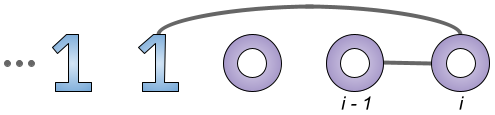The two observations lead to a dynamic programming solution. Let $$dp[i][j]$$$ be the optimum time to collect the first $$i$$$$$0$$$⁠-shaped balls and the first $$j$$$$$1$$$⁠-shaped balls. The base case is $$dp=0$$$. For $$i+j>0$$$, suppose again that the rightmost of these $$i+j$$$balls is $$0$$$⁠-shaped and it has the coordinate $$x$$$. The case when the rightmost ball is $$1$$$⁠-shaped is symmetric. To eliminate some other corner cases, $$dp=2x$$$, $$dp[i]=\min(dp[i-1],dp[i-2]+\mathbf{C})+2x$$$ for $$i \ge 2$$$, and $$dp[j]=dp[j-1]+2x$$$ for $$j \ge 1$$$. For the general case with $$i \ge 2$$$ and $$j \ge 1$$$, if the penultimate ball is $$1$$$⁠-shaped, then $$dp[i][j]=dp[i-1][j-1]+2x$$$(Observation 1). Otherwise, we can choose to match the last $$0$$$⁠-shaped ball with the previous $$0$$$⁠-shaped ball or the rightmost $$1$$$⁠-shaped ball (Observation 2), namely, $$dp[i][j]=\min(dp[i-2][j]+\mathbf{C},dp[i-1][j-1])+2x$$$. The final answer is $$dp[N_0][N_1]$$$, where $$N_0$$$and $$N_1$$$ denote the total number of $$0$$$⁠-shaped and $$1$$$⁠-shaped balls, respectively. The time complexity of this algorithm is $$O(\mathbf{N}^2)$$$. ### Test Set 2 Using dynamic programming from a different angle, we can solve the problem in linear time, apart from the initial sorting. Let $$dp[i]$$$ be the optimum time to collect the first $$i$$$balls. As the base cases, $$dp=0$$$ and $$dp=2\mathbf{X_1}$$$. To calculate $$dp[i]$$$ for $$i \ge 2$$$, suppose once more that the $$i$$$-th ball is $$0$$$⁠-shaped. If the $$(i-1)$$$-th ball is $$1$$$⁠-shaped, we can match the last two balls and $$dp[i]=dp[i-2]+2\mathbf{X_i}$$$ (Observation 1). Otherwise, using Observation 2, we have the options to match the last two $$0$$$⁠-shaped balls and collect all balls in $$dp[i-2]+\mathbf{C}+2\mathbf{X_i}$$$ seconds, or to match $$i$$$-th ball with the rightmost $$1$$$⁠-shaped ball $$j$$$. The dynamic programing recurrence is not obvious in the latter case, though, as we do not know the optimum matching for the first $$i-1$$$ balls except for ball $$j$$$. What happens to the $$0$$$⁠-shaped balls in-between $$j$$$and $$i$$$? We are missing another key observation here.

Observation 3: If there is an optimal matching of the first $$i$$$balls such that the $$0$$$⁠-shaped ball $$i$$$is matched with the rightmost $$1$$$⁠-shaped ball $$j$$$and $$i-1 \neq j$$$, then the $$0$$$⁠-shaped ball $$i-1$$$ is not matched with another $$0$$$⁠-shaped ball. Proof: Assume on the contrary that we have two pairs of matched balls $$(i,j)$$$ and $$(i-1,k)$$$, $$k \lt i-1$$$, such that ball $$k$$$is $$0$$$⁠-shaped. These two matched pairs contribute $$2\mathbf{X_i}+2\mathbf{X_{i-1}}+\mathbf{C}$$$seconds to the overall matching cost. But then we can rearrange the matchings as $$(i,i-1)$$$ and $$(j,k)$$$costing us only $$2\mathbf{X_i}+\mathbf{C}+2\times\max(\mathbf{X_j},\mathbf{X_k})$$$ seconds, which is $$2(\mathbf{X_{i-1}}-\max(\mathbf{X_j},\mathbf{X_k}))$$$seconds less. This contradicts the optimality assumption of the given matching. It follows from Observation 3 that the $$0$$$⁠-shaped ball $$i-1$$$must be matched with another $$1$$$⁠-shaped ball, specifically the rightmost unmatched $$1$$$⁠-shaped ball. And we can extend this argument and repeatedly match $$0$$$⁠-shaped balls with $$1$$$⁠-shaped balls sweeping leftward for as long as there is another $$0$$$⁠-shaped ball to the right of a matched $$1$$$⁠-shaped ball. This process is ilustrated in the drawing below.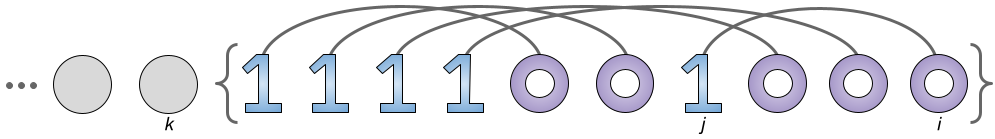Let $$k$$$ be the rightmost unmatched ball after the above $$0$$$⁠-⁠$$1$$$ matching process. There are no shape changes in the set of balls $$\{k+1,k+2,\ldots,i\}$$$and the cost of collecting those balls is twice the sum $$X_\mathrm{0-shaped}(k+1,i)$$$ of $$x$$$-coordinates of $$0$$$⁠-shaped balls in $$\{k+1,k+2,\ldots,i\}$$$. Therefore, the cost of collecting all $$i$$$ balls in this way is $$dp[k]+2\times X_\mathrm{0-shaped}(k+1,i)$$$. $$X_\mathrm{0-shaped}(k+1,i)$$$ can be calculated in $$O(1)$$$time using prefix sums. But how do we get the index $$k$$$ efficiently without actually carrying out the matching process? Note that $$k$$$is the largest index such that $$k \lt i$$$ and the set $$\{k+1,k+2,\ldots,i\}$$$contains equal number of $$0$$$⁠-shaped and $$1$$$⁠-shaped balls. Consider the balance $$b_i$$$ of 0/1 balls at each index $$i$$$, namely, $$b_i=z_i-o_i$$$, where $$z_i$$$and $$o_i$$$ is the number of $$0$$$⁠-shaped and $$1$$$⁠-shaped balls in the set $$\{1,2,\ldots,i\}$$$. The set $$\{k+1,k+2,\ldots,i\}$$$ has equal number of $$0$$$⁠-shaped and $$1$$$⁠-shaped balls if and only if $$b_k=b_i$$$. The index $$k$$$ can be looked up in $$O(1)$$$time if we maintain a hash-table of indices, when each balance was last registered. If the current balance $$b_i$$$ is seen for the first time, it means that there are not enough $$1$$$⁠-shaped balls to match all $$0$$$⁠-shaped balls with, and we can choose $$k=0$$$. We are performing a constant number of operations at each index in this approach, so the overall time complexity is dominated by the sorting, thus $$O(\mathbf{N} \log \mathbf{N})$$$.

Test Data
info We recommend that you practice debugging solutions without looking at the test data.